### Home > MC2 > Chapter Ch7 > Lesson 7.1.5 > Problem7-48

7-48.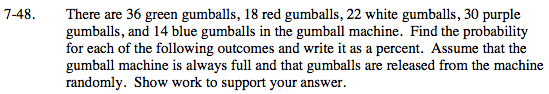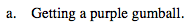The probability of getting a purple gumball is the number or purple gumballs divided by the total number of gumballs.

$\frac{30}{120} = 25$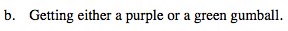The probability of getting either a purple or a green gumball is the sum of the probability of getting a purple gumball and the probability of getting a green gumball.

$\text{P(purple) + P(green)} = \frac{30}{120} + \frac{36}{120} = \frac{66}{120} = 55$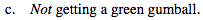What is the probability of getting a green gumball?

Since you can either get a green gumball or not get a green gumball, the probability of not getting a green gumball is 1 (or 100%) minus the probability of getting a green gumball.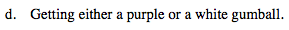What is the probability of getting a purple gumball?
A white gumball?

See (b).